## The contents

A direct interconnection network (IN) of a multiprocessor system is represented by a connected graph whose vertices represent processing nodes and edges represent communication links. A processing node (PN) usually consists of one or more processors, local memory, and communication router. This section is devoted to the description and analysis of topologies and properties of important INs.

 Back to the beginning of the page Back to the CS838 class schedule

## Basic notions and terminology

### Alphabets and strings

d-ary alphabet
is denoted by Zd={0,1,..,d-1}. The operation + modulo n is denoted by +n.
n-letter d-ary strings
Zdn={xn-1... x0; xi in Zd}, n>= 1. The length of string x is denoted by len(x). The empty string (i.e., of length 0) is denoted by e. The i-fold concatenation of string x is denoted by xi.
Binary alphabet
is denoted by B. The inversion of bit bi is \non(bi)=1-b1. If b=bn-1.. bi+1bibi-1.. b0 in Bn, then \noni(b)=bn-1.. bi+1\non(bi)bi-1.. b0.

### Graph theory

Vertex and edge set
of graph G is denoted by V(G) and E(G), respectively. Two adjacent vertices u and v form edge ( u,v). They are incident with the edge. Edges ( u,v) and ( v,w) are adjacent.
H = subgraph
of G, H\subset G, if V(H)\subset V(G) and E(H)\subset E(G).
H = induced subgraph
of G if it is a maximal subgraph of G with vertices V(H).
H = spanning subgraph
of G if V(H)=V(G).
Union
of two disjoint graphs G1 and G2, G1\cup G2, is a graph with vertices V(G1)\cup V(G2) and edges E(G1)\cup E(G2).
Cartesian product
of G1 and G2 is graph G=G1 x G2 with V(G)={(x,y); x in V(G1), y in V(G2)} and E(G)={( (x1,y),(x2,y)); ( x1,x2) in E(G1)}\cup {( (x,y1),(x,y2)); ( y1,y2) in E(G2)}.CAPTION: An example of a cartesian product

Degree of vertex
u, degG(u), is the number of neighbors of u.
Degree set of graph
G, deg(G), is the set {degG(u); u in V(G)}.
Maximum degree
of G is \triangle(G)=max(deg(G)).
Minimum degree
of G is \delta(G)=min(deg(G)).
k-regular graph
G has \triangle(G)=\delta(G)=k.
Connected graph
Every pair u, v of vertices is joined by a path P(u,v), a sequence of adjacent edges.
Path length
len(P(u,v)), is the number of edges P(u,v).
Distance
between vertices u and v, distG(u,v), is the length of a shortest path joining u and v.
Average distance
in G, distG(u,v), is \Sigmau,vdistG(u,v)/(N(N-1)).
Diameter
of G, diam(G), is the maximum distance between any two vertices of G.
Cycle
is a closed path.
Vertex-disjoint paths
P1(u,v) and P2(u,v) have no vertices in common except for u and v
Edge-disjoint paths
P1(u,v) and P2(u,v) have no edges in common.
Connectivity
(Vertex) connectivity of G, \kappa(G), is the minimum number of vertices whose removal results in a disconnected graph. Edge connectivity of G, \lambda(G), is the minimum number of edges whose removal results in a disconnected graph. It follows that
\kappa(G)<= \lambda(G)<=\delta(G).CAPTION: Example of a graph G with \kappa(G)=1 and \lambda(G)=\delta(G)=2.

k-connected graph
has \kappa(G)=k. Similarly, k-edge-connected graph has \lambda(G)=k.
Optimal connectivity
\kappa(G)=\lambda(G)=\delta(G).
Menger's theorem
Between any two distinct vertices of G, there are at least \kappa(G) vertex-disjoint paths and at least \lambda(G) edge-disjoint paths.
Fault diameter
of a connected graph G is the maximum over the lengths of all the shortest vertex-disjoint paths between any two vertices in G. Similarly, we can define the fault distance between any two vertices.
Bipartite graph
There exists a bipartition (or 2-coloring) of its vertex set V, which is a partitioning of V into two disjoint subsets V1 and V2 such that each edge of G has one vertex in V1 and the other vertex in V2.
Balanced bipartite graph
has a bipartition (V1,V2) with |V1|=|V2|.CAPTION: Examples of bipartite graphs, the first one is not balanced

Hamiltonian path/cycle
of a connected G is a path/cycle joining all vertices of G. A graph having a hamiltonian cycle is hamiltonian. A bipartite graph can have a hamiltonian cycle only if it is balanced.
(Edge) bisection width
of G, bwe(G), is the smallest number of edges removal of which divides G into two parts of equal size (up to one vertex).
Vertex bisection width
of G, bwv(G), is the smallest number of vertices removal of which divides G into two parts having at most [|V|/2] vertices each.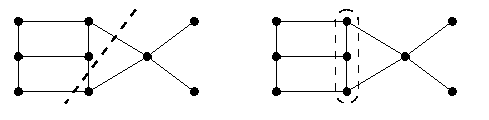CAPTION: An example of a graph with both edge and vertex bisection width 3

Isomorphism
Two graphs are isomorphic if they can be made identical by relabeling vertices.
Automorphism
of G is any isomorphic mapping of G to itself.
Vertex-symmetric
graph looks the same independently of from which vertex you look at it, i.e., for any two distinct vertices u and v, there is an automorphism of the graph sending u to v.
Edge-symmetric
graph looks the same independently of from which edge you look at it, i.e., for any two distinct edges e1 and e2 in E(G), there is an automorphism of G sending e1 to e2. Graph G on Figure is edge symmetric, but it is not vertex symmetric. It becomes vertex symmetric if the vertices 1 and 3 are merged. A vertex symmetric graph must be regular.
Digraphs = Oriented graphs
A digraph G has arc set A(G). Arc ( u->v) is incident from u to v u,v in V(G). Vertex u (v) is incident from (to) arc ( u->v), respectively. Arcs ( u->v) and ( v->w) are adjacent. The in-degree and out-degree of u in V(G), denoted by \indegG(u) and \outdegG(u), is the number of arcs incident to and incident from u, respectively. The other notions are derived similarly, digraphs have oriented paths (dipaths), oriented diameters, strong connectedness, and strong connectivity.
While describing INs, we will often use terms nodes and links (or channels) instead of vertices and edges, terms input (output) links or channels, respectively, instead of arcs incident from (incident to) a vertex. We will often use the terms vertices/nodes or edges/links interchangeably. All logarithms are binary if not stated otherwise.
 Back to the beginning of the page Back to the CS838 class schedule

## Requirements on interconnection networks

An IN should transfer a maximum number of messages in the shortest time with minimum cost and maximal reliability. Clearly, any design of an IN is a tradeoff of various contradictory requirements. The most important requirements are the following:
Small diameter and small average distance
Small average distance allows small communication latency, especially for distance sensitive routing, such as store-and-forward. But it is also crucial for distance insensitive routing, such as wormhole routing, since short distances imply less used links and buffers, and therefore less communication contention.
Small and fixed vertex degree
Small and constant vertex degree allows simple and low-cost universal (i.e., independent of the network size) routers which amortizes the design costs. On the other hand, it implies less links and lower connectivity and larger distances. Ideally, we would like to get low constant vertex degree and at most logarithmic diameter simultaneously. Given N-vertex graph such that \triangle(G)<= k for some constant k, the number of vertices reachable in G within i steps from any vertex is O(ki). Hence, N=O(k^diam(G)), which is equivalent to diam(G)=\Omega(log N). Fixed-degree networks cannot have better than logarithmic diameter.
Large bisection width
Many problems can be solved in parallel using binary divide-and-conquer: split the input data set into two halves and solve them recursively on both halves of the IN in parallel, and then merge the results in both halves into the final result. Small bisection width implies low bandwidth between both halves and it can slowdown the final merging phase. On the other hand, a large bisection width is undesirable for a VLSI design of the IN, since it implies a lot of extra chip wires.
High connectivity and fault tolerance
The network should provide alternative paths for delivering messages in case of link or node faults or communication congestions. Large packets can be delivered faster if they can be split into smaller chunks sent along disjoint paths.
Small fault average distance and diameter
To have these alternative or parallel disjoint paths as short as possible, small fault average distances and small fault diameter are naturally desirable.
Hamiltoniaty
The existence of at least a hamiltonian path is not the most important requirement, but it is useful whenever we need to label processors with numbers 1,...,p so that adjacent processors get successive numbers (for example in sorting algorithms).
Hierarchical recursivity
INs are usually defined using some independent parameters, called dimensions. The set of all graphs of different dimensions based on a given definition forms a topology. A topology is hierarchically recursive if a higher-dimensional graph contains lower-dimensional graphs with the same topology as subgraphs. Many INs used in parallel systems are hierarchically recursive, topologies based on cartesian product are one example. Recursiveness makes the design and technology of manufacturing INs easier. Large scale problems that can be solved in parallel by recursive decomposition into smaller subproblems can be easily and efficiently mapped on the hierarchically recursive topologies using induction.
Incremental extendability and incremental scalability
If the definition of the topology allows graphs of any size, the topology is said to be incrementally extendable. There are incrementally extendable topologies, and some are at least partially extendable, since they allow size granularity only greater than 1. Some hierarchically recursive topologies allow graphs of specific discrete sizes, such as powers of two. If a topology is incrementally extendable, a very important question is how the structure of an instance of size n differs from the structure of an instance of size n+k for some integer constant k>=1. An (n+k)-vertex instance can be obtained from a n-vertex one by removing r(k) edges (to get a subgraph of the larger instance) and by adding additional vertices and corresponding edges to this subgraph. If r(k)=O(k), the topology is said incrementally scalable. Very few topologies are incrementally scalable. For example, 2-D meshes are incrementally extendable, but not scalable.
Symmetry
This is a very important requirement. Many IN topologies are vertex or edge symmetric. Intuitively, a symmetric network is easy to understand and the design of parallel and communication algorithms is very much easier, since it is irrelevant where the computation and/or communication starts or in which directions it will evolve. Also, the symmetry is helpful for solving issues related to VLSI design.
Support for routing and collective communication
This is a crucially important property and we will devote it several lectures. The network topology should enable an simple shortest path routing, so that the basic routing algorithm could be implemented in hardware. Equally important parameters of an IN are complexities of permutation routing and one-to-all and all-to-all communication operations. The design of efficient communication algorithms is very simplified if the topology is symmetric and it also depends on the communication technology and router architecture.
Embeddability of and into other topologies
The efficiency of a parallel algorithm running on a parallel machine depends on the similarity between the process graph and the underlying physical IN topology. We need a suitable mapping or embedding of the process graph into the topology. Desirable are those topologies which are able to simulate efficiently other topologies.
Simple VLSI or 3-D layout
Any VLSI implementation of an IN implies VLSI-related requirements, such as easy mapping to rectangular grid, decomposition of a large IN into building blocks so that the lengths and numbers of inter-chip wires is minimal (recall the bisection width above). Except for the bisection width, the discussion of VLSI design issues is beyond the scope of this course.
In the rest of the section, we give a survey of definitions and properties of several well-known topologies for INs. Some topologies are understood better than some others so that the description of properties will vary for various topologies.
 Back to the beginning of the page Back to the CS838 class schedule

## Mesh-based topologies

This family of topologies, also called strictly orthogonal, include hypercubes, meshes, and tori. These are the most important interconnection topologies due to their simplicity and they are also the best understood topologies.

### Binary hypercube of dimension n, Qn

The n-dimensional binary hypercube (or n-cube) is a graph with 2n vertices labeled by n-bit binary strings, with edges joining two vertices whenever their labels differ in a single bit (see Figure).CAPTION: Hypercube Q4

 V(Qn)=Bn |V(Qn)|=2n E(Qn)={( x,\noni(x)); x in V(Qn),0<= i
• The hypercube is a regular balanced bipartite hamiltonian graph.
• The distance between two vertices u and v in Qn is the Hamming distance.
• It has logarithmic diameter. The average distance in Qn is n/2.
• It is not a constant-degree topology, the vertex degree grows logarithmically with the number of vertices. If the number of nodes of a parallel computer doubles, the degree of each vertex increases by one. A router with k communication ports can be used for building a machine with at most 2k nodes. This problem is solved by constant-degree and logarithmic-diameter derivatives of the hypercube, called hypercubic networks, see Page.
• The hypercube has the largest possible bisection width. Each vertex in one half is adjacent to its image in the other half.
• The connectivity of Qn is n, which is optimal. If k<= n is the distance between vertices u and v, then there are n vertex-disjoint (therefore edge-disjoint) paths between u and v such that k of these paths have length k and n-k paths have length k+2.
• The fault diameter of Qn is n. If we remove n-1 edges from Qn, there still exists a path of length at most n between any two vertices.
• Fault tolerance is also excellent, there are k! different shortest paths between two vertices in distance k, since k! is the number of permutations of k coordinates in which the addresses differ.
• The hypercube is hierarchically recursive. Qn is a cartesian product of subcubes:
Qn=Qk x  Qn-k,  k in {1,...,n-1}.

There are n\choosek ways how to make this decomposition. In particular, for any j in N there exists a canonical decomposition of Qn into two (n-1)-cubes Qn-1[j=0] and Qn-1[j=1] (This gives the optimal bisection width.) For example, since Q6=Q3 x Q3, we can view a Q6 as a 3-cube, whose vertices are 3-cubes (Figure shows a simplified picture of that). But also Q6=Q2 x Q4=Q2 x Q2 x Q2, and so on. Each k-dimensional subcube S of Qn is uniquely specified by its address sn-1... s1s0, where si in {0,1,*}, with exactly k appearances of the don't care symbol *. 0-dimensional subcubes are vertices and subcubes are generalized internally structured" vertices. Vertices correspond to minterms and subcubes to terms in Boolean algebra.
• The hypercube topology is not incrementally extendable, it is defined only for powers of two. However, many incrementally extendable generalizations have been proposed, such as incomplete hypercubes.
• The hypercube is both vertex- and edge-symmetric graph. The automorphism group of Qn consists of 2n x n! translations and rotations. A translation is a systematic renaming of vertices. Let \XOR denote the bitwise XOR operation. Translation from u to v is a mapping for all x in V(Qn) (x-> (u\XOR v)). A rotation is a permutation (renaming) of dimensions.
• The basic routing algorithm is trivial and optimal, usually hardwired, and called e-cube routing: The bits in which the sender and receiver differ are compared in a fixed order, usually left to right.
• There exist optimal algorithms for collective communication in almost all communication models.
• The hypercube can also simulate efficiently almost any other topology.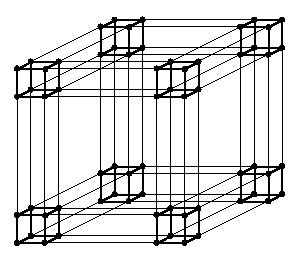CAPTION: Q6=Q3 x Q3.
The notion of subcubes is extremely important for using a hypercube multiprocessor as a multiuser multitasking machine. Users declare the numbers of required nodes for their tasks and the operating system allocates, if possible, subcubes of the appropriate size, which are released upon completion of the tasks. This is called the subcube allocation problem and there exist many algorithms for it which must maintain lists of allocated and free subcubes. Dynamic allocation and releasing of subcubes lead to the fragmentation problem in the hypercube similar to segment fragmentation of dynamic or virtual memory. The hypercube is the best topology for implementing most parallel and communication algorithms. It serves as a testbed for parallel feasibility of problems on distributed memory architectures. Its only drawback is the logarithmic vertex degree and lack of scalability. Several families of older massively parallel multiprocessors were based on the hypercube: nCUBE series 1 and 2, Intel's iPSC/2 and iPSC/860, TMC's CM-2, and several others. Currently, SGI Origin's interconnection network uses hypercube topology.

### n-dimensional mesh of dimensions z1,z2,..,zn, M(z1,z2,..,zn)

Given integers zi>= 2, 1<= i<= n, the n-dimensional mesh with side lengths zi, M(z1,z2,..,zn), is defined as follows (Figure(a) shows mesh M(3,3,4)).
 V(M(...))={(a1,a2,..,an) ; 0<= ai<= zi-1 for all i in {1,..,n}} |V(M(...))|=\prodi=1n zi E(M(...))={( (..,ai,..),(..,ai+1,..)); 0<= ai<= zi-2} |E(M(...))|=\sumi=1n(zi-1)\prod_j=1\atop j\not=in zj diam(M(...))=\sumi=1n(zi-1)=\Omega(\root n \of |V(M(...))|) deg(M(...))={n,..,n+j}, j=|{zi; zi>2}| bwe(M(...))=(\prodi=1n zi)/maxizi if maxizi is even,                          \Omega((\prodi=1n zi)/maxizi) otherwise.
• When dealing with a mesh, we usually assume that its dimension n is fixed. If we want to change its size, we change the side lengths. All the properties explained in what follows are stated under the assumption of fixed mesh dimension.
• The most practical meshes are, of course, 2-D and 3-D ones: they have trivial VLSI or 3-D design (2-D meshes are planar) and allow simple and natural mapping of 2-D and 3-D space problems with locality of interprocessor interactions (computer graphics and virtual reality, fluid mechanics, modeling of 3-D systems in earth sciences or astronomy, etc). They are also natural, even though not optimal, topologies for linear algebra problems (parallel solvers of linear or differential equations). 1-D meshes are called linear arrays and they are incrementally scalable.
• The mesh M(k,k,..,k) is called k-ary n-cube.
• A mesh is a bipartite (not necessarily balanced) graph. If at least one side has even length, the mesh has a hamiltonian cycle. A hamiltonian path exists always.
• Meshes are not regular, but the degree of any vertex is bounded by 2n. Of course, the degree of a corner vertex is less than the degree of an internal vertex.
• Therefore, meshes are not vertex symmetric. This complicates the design of some parallel and communication algorithms, but on the other hand, there are problems taking advantage of the asymmetry between boundary and internal vertices, e.g., parallel finite difference schemes for solving differential equations in 2-D and 3-D space.
• The distance between two vertices is the sum of differences in corresponding coordinates.
• The diameter is n-th root of the size of graph. It is large for lower-dimensional meshes, and therefore, they are not optimal for algorithms with global interactions, e.g., parallel sorting, or problems where global broadcasting or permutations are required, such as in parallel solvers of linear equations. So, 2-D or 3-D mesh are simple extendable INs with small fixed vertex degree.
• The exact value of bisection width is known only if maxizi is even. Then removing the middle edges of linear paths in the longest-side direction results in a bisection of minimal size. However, if maxizi is odd, the exact value of the bisection width is not known for n>= 4. However, it is easy to calculate it for 2- or 3-D meshes. For example, bwe(M(11,9,6))=64. (Can you show that?) Lower-dimensional meshes have very low bisection width, which creates a bottleneck for many parallel mesh-based algorithms.
• The connectivity is optimal. The proof is similar to that for hypercubes. Also, the shortest path redundancy is optimal.
• The fault diameter is optimal, equal to the nonfaulty diameter. Fault distances are by at most 4 larger than nonfaulty ones.
• Meshes are also hierarchically recursive. They can be decomposed into cartesian products of lower-dimensional meshes, up to the canonical decomposition into a cartesian product of linear arrays: M(z1,z2,..,zn)=M(z1,z2,.., zn-1) x M(zn)=M(z1,..,zi) x M(zi+1,..,zj) x M(zj+1,..,zn)=M(z1) x M(z2) x ... x M(zn). Submeshes are also denoted by strings with don't-care symbols.
• Meshes are incrementally extendable, but not incrementally scalable. You can define a mesh of any size, but the amount of edges that must be removed from a smaller one to construct a larger one, can be very large.
• The basic shortest-path routing in general meshes is also trivial. It is called dimension ordered routing. Again, addresses of the sender and receiver are inspected in a prespecified order and the message is sent via the first port corresponding to a coordinate in which the addresses differ. In 2- and 3-D meshes, this is called XY and XYZ routing.
• Many optimal communication and parallel algorithms have been developed for meshes (optimal in the sense of matching the mesh lower bounds due to large diameter, low bisection width, etc), namely for 2-D and 3-D meshes. Many of them will be treated in detail in this course.
• Besides standard meshes, various modifications have been proposed, such reconfigurable meshes, meshes of buses, and so on. We will not deal with these variants here.
The most important mesh-based parallel computers are Intel's Paragon (2-D mesh) and MIT J-Machine (3-D mesh). Also transputers used 2-D mesh interconnection. Processors in mesh-based machines are allocated by submeshes and the submesh allocation strategy must handle possible dynamic fragmentation and compaction of the global mesh network, similarly to hypercube machines.CAPTION: Mesh M(3,3,4)

### n-dimensional torus of dimensions z1,z2,..,zn, T(z1,z2,..,zn)

Given integers zi>= 2, 1<= i<= n, the n-dimensional torus T(z1,z2,..,zn), also called toroidal or wrapped mesh, with side lengths z1,z2,..,zn, is defined as follows.
 V(T(...))=V(M(...)) |V(T(...))|=\prodi=1n zi E(T(...))={( (..,ai,..),(..,ai+_zi1,..)); 0<= ai
Figure(b) shows a 3-dimensional torus T(3,3,4). Adding wrap-around edges changes dramatically many structural properties, even though many feature remain similar to meshes. We will highlight some of them.
• A 1-dimensional torus is simply a cycle or ring.
• The torus T(k,k,..,k) is called k-ary n-torus.
• Tori are bipartite iff all side lengths are even. But then, they are balanced.
• Any torus has a hamiltonian cycle.
• Tori are regular and vertex symmetric. The automorphism group consists of translations. k-ary n-tori are also edge symmetric and have rotations as automorphisms.
• The diameter and average distance of a torus drops to nearly one half compared to a mesh with the same dimensionality, and the connectivity and the bisection width doubles. Hence, the connectivity of n-dimensional torus, 2n, is also optimal.
• They are also hierarchically recursive, again thanks to cartesian product structure. Each torus can be canonically decomposed into a cartesian product of cycles.
• Scalability properties are even slightly worse here than at meshes.
• Cyclic structure of tori makes shortest path routing algorithms more complicated, but the basic approach remains dimension ordered routing.
• Similarly to meshes, many fundamental problems are known to have topologically optimal solutions on tori and we will demonstrate many of them here.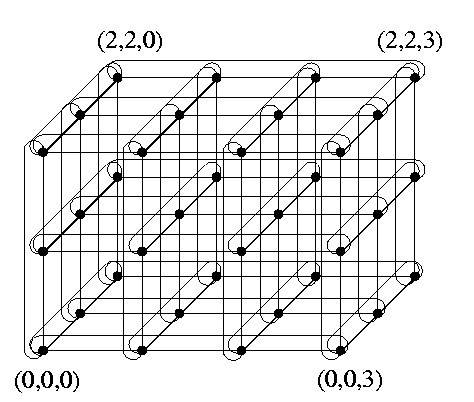CAPTION: Torus T(3,3,4).

### Comparisons of hypercubes, meshes, and tori

• M(2,2,..,2) is isomorphic Qn
• n-dimensional meshes and tori are generalizations of Qn
• For some k and n, k<n, a k-dimensional mesh/torus can be a subgraph of Qn.
%See Chapter \refEmbeddingsChapter for more details. For example, Figure illustrates that torus T(4,4) is isomorphic to Q4.CAPTION: T(4,4)= Q4.
Table shows the tradeoff between the diameter, number of edges, and bisection width of M(8,8,4), T(8,8,4), and Q8. All these graphs have the same size |V()|=256 and M(8,8,4)\subset T(8,8,4)\subset Q8.

 M(8,8,4) T(8,8,4) Q8 diam() 17 10 8 |E()| 640 768 1024 bwe() 32 64 128
CAPTION: Comparison of several characteristics of meshes, tori, and hypercubes.
The tori are likely to become widely used interconnection networks. For example, Cray/SGI T3D and T3E and Convex Exemplar are based on 3-D torus, Intel/CMU iWarp uses 2-D torus, and several commercial machines use rings as a primary interconnect.
 Back to the beginning of the page Back to the CS838 class schedule

## Hypercubic topologies

Hypercubic networks are logarithmic-diameter and constant-degree derivatives of the hypercube. The price to be paid for the constant vertex degree is worse extendability: the size of instances becomes n2n or similar. Therefore, they grow even faster with increasing dimension than the hypercube. They share many properties, such as bisection width \Omega(N/log N). Let us shortly describe two representants: cube-connected cycles and butterflies.

### Cube-connected cycles of dimension n, CCCn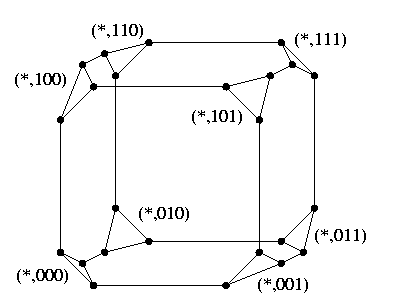CAPTION: Cube Connected Cycles CCC3.

 V(CCCn)={(i,x) ; 0<= i3, diam(CCC3)=6 deg(CCCn)={3} bwe(CCCn)=2n-1
CCCn is constructed from Qn by replacing each hypercube vertex with a cycle of n vertices so that each vertex takes care of just one hypercube direction. See Figure where (*,x) stands for {(0,x), (1,x), (2,x)}. Figure shows other possible ways how to view CCC topology. Part (b) is a useful simplification, CCC is viewed as a pancake made of all cycles, all the cycles look alike, and all hypercube edges are projected into vertex loops.
• CCCn is balanced bipartite iff n is even.
• Any CCCn has a hamiltonian cycle.
• CCC is a regular and vertex-symmetric topology. Figure(b) shows it clearly. It is not an edge-symmetric graph, there are two kinds of edges: hypercube edges and cycle edges.
• It has optimal connectivity 3. Between two arbitrary vertices, there are at most two shortest paths.
• Shortest path routing is based on e-cube routing and Figure(b) is helpful in understanding it.
• In contrast to the hypercube, CCC is not hierarchically recursive. We can split a CCCn into two halves which are very similar to CCCn-1, but they have one abundant vertex in each cycle.CAPTION: Another view of CCC.

### Butterfly of dimension n

Actually, there are two kinds of butterfly networks, wrapped and ordinary. The wrapped butterfly, wBFn, is defined as follows:
 V(wBFn)={(i,x); 0<= i
If each cycle of wBFn shrinks to a single vertex and redundant edges are removed, we get Qn (see Figure). Wrapped butterfly topology has basically the same properties as CCC, except that it has more edges, and therefore larger bisection width and lower diameter.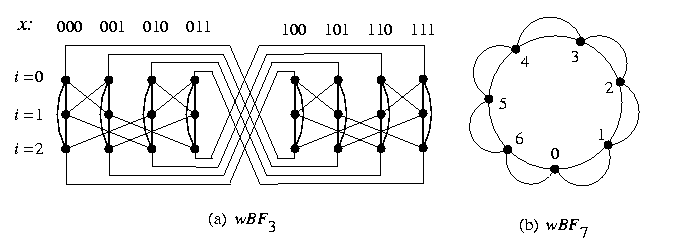CAPTION: Wrapped butterflies
An n-dimensional ordinary butterfly, oBFn, can be made form wBFn by replacing n-vertex cycles with (n+1)-vertex paths (or in other words, an ordinary butterfly is a hypercube with each vertex unfolded into a path).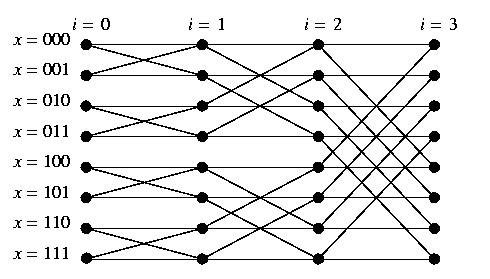CAPTION: Ordinary butterfly oBF3.

 V(oBFn)={(i,x); 0<= i<= n \wedge x in Bn} |V(oBFn)|=(n+1)2n E(oBFn)={((i,x),(i+1,x)), ((i,x),(i+1,\noni(x)))\mid(i,x) in V(oBFn),i
• The vertices of oBFn are organized into columns (stages) i, 0<= i<= n, and rows x, 0<= x<= 2n-1. There are also two kinds of edges in the ordinary butterfly: straight edges and cross (hypercube) edges (see Figure). Edges linking vertices of stages i and i+1 are said to be of stage i.
• The ordinary butterfly is not vertex symmetric, it is not even regular.
• It is not hamiltonian.
• On the other hand, it is hierarchically recursive: oBFn contains two oBFn-1 as subgraphs.
• The connectivity is 2, which is optimal.
• There exists only one shortest path between any pair of vertices (0,x) and (n,y). The path traverses each stage exactly once using a cross edge of stage i iff the row numbers x and y differ in the i-th bit. It follows that the basic shortest-path routing is basically the e-cube routing from hypercubes, see Page.
 Back to the beginning of the page Back to the CS838 class schedule

## Tree-based topologies

Process graphs have often tree-like structure. However, the bottleneck at the root and poor connectivity and robustness disqualifies any simple complete tree as a potential general purpose IN topology. Fortunately, tree-like graphs can be efficiently mapped on most usual INs. The only commercially important tree-based parallel machine have been TMC's CM-5 with its famous fat tree and some special-purpose pyramidal machines. In this course, we will describe an interesting hybrid of meshes and trees.

### 2-D mesh of trees of height n, MTn

2-D and 3-D meshes suffer from large diameter, whereas trees suffer from small connectivity and bisection width. 2-D mesh of trees is a hybrid topology based on the 2-D mesh and complete binary tree, which has the diameter of the tree topology (i.e., logarithmic) and the bisection width of the 2-D mesh topology (i.e., square root of the size). For integer n>= 1, let N=2n. Then MTn is defined as follows: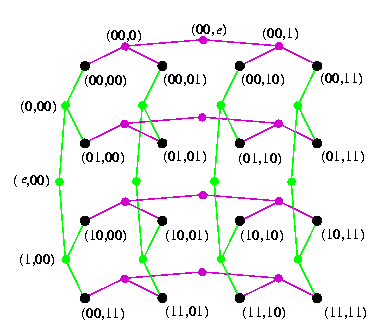CAPTION: Mesh of trees MT2.

 V(MTn)=(Bn x \cupi=0nBi)\cup(\cupi=0nBi x Bn) |V(MTn)|=3N2-2N E(MTn)={((x,y),(xa,y)); len(x)< n\wedge a in B} |E(MTn)|=4N(N-1) \cup {((x,y),(x,ya)); len(y)< n\wedge a in B} bwe(MTn)=N=\Theta(\sqrt|V(MTn)|) deg(MTn)={2,3} diam(MTn)=4log N=4n
• The mesh-of-trees topology can be defined for any mesh M(2k,2l). It can be even defined for any n-dimensional mesh with side lengths equal to powers of two. We will discuss only the 2-D square case for simplicity.
• Informally, a mesh-of-trees is a 2-D mesh whose linear rows and columns are replaced with row and column complete binary trees of height n, CBTn. The original mesh vertices are leaves in the intersections of the row and column trees and the additional vertices are exactly the internal vertices of the trees. It follows that MTn\subsetCBTn x CBTn.
• An N-vertex mesh-of-trees is constant vertex degree O(log N)-diameter and \Omega(\sqrtN)-bisection width topology.
• The mesh-of-trees topology is bipartite, but not balanced, therefore it is not hamiltonian.
• It is hierarchically recursive. By removing row- or column-tree roots, it decomposes into disjoint submeshes-of-trees. Low-level submeshes-of-trees can be used for local computations and the root nodes can be used for global communication and coordination.
• It is neither regular neither vertex symmetric.
• Its extendability is rather poor.
• Shortest-path routing is simple, it alternates routing in 2-D meshes and trees. Assume source vertex u and destination vertex v.
• If u is in a row tree and v in a column tree, the shortest path from u to v is uniquely defined via the intersection leaf of both trees.
• If u and v are in two different row trees Tu and Tv, compare the level of u and v in their trees. If u is lower than v, then go down in Tu to any leaf in the subtree, use the corresponding column tree to get to Tv, and use the tree routing in Tv to get to v (hence pass through the root of the smallest common subtree of v and of the homothetic image of u in Tv). If u is higher than v, then go first to the image of v in Tu and via any leaf get to Tv then. The number of different shortest paths depends on the heights of the vertices.
• The routing between two internal nodes of column trees is similar.
No commercial machine has been built upon this topology yet.
 Back to the beginning of the page Back to the CS838 class schedule

## Shuffle-based topologies

The adjacency in orthogonal and hypercubic topologies is based on incrementing and/or inverting coordinates. In shuffle topologies, the adjacency is based on shifting the whole strings, typical operation is left shift and left rotation by one position, which enforces orientation on edges. Since most of notions and results apply equally to both directed and undirected versions of these topologies and digraphs are regular in contrast to undirected graphs, we will concentrate on digraphs only. Shuffle-based topologies have very rich, but also rather complicated structure. In general, they are more difficult to understand compared to previous topologies. They have the following properties:
• Logarithmic diameter and constant vertex degree.
• Optimal or nearly optimal connectivity.
• Fault diameter nearly the same as non faulty one, similarly for fault average distances.
• Large bisection width of order N/log N.
• Simple shortest-path routing.
• They are neither symmetric nor hierarchically recursive.
• They are rich of cycles of various lengths, since the rotation induces equivalence classes of vertices called necklaces.
• At the same time, they are rich of subtrees and spanning trees, since the general shift operation induces subtrees.
The shuffle-based family includes three main topologies, shuffle exchange, de Bruijn, and Kautz, and their variations. We will describe only the first two main ones.

### Shuffle-exchange networks

Even if the shuffle-exchange topology can be defined for any alphabet, the focus of computer science has always been primarily on the binary shuffle-exchange topology. The shuffle of a binary string x, \sigma(x), is its left rotation by one bit. The inversion of the least significant bit of x is called the exchange operation. Shuffle-exchange digraph of dimension n, SEn, is defined as follows: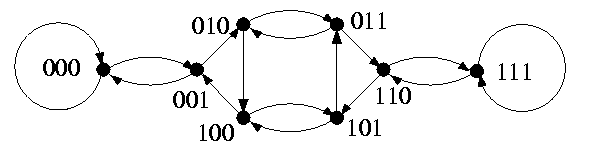CAPTION: Digraph SE3.

 V(SEn)=Bn |V(SEn)|=2n A(SEn)={( x->\non0(x)); x in Bn}\cup{( x->\sigma(x)); x in Bn} |A(SEn)|=2n+1 in deg(SEn)=\outdeg(SEn)={2} diam(SEn)=2n-1 bwe(SEn)=\Theta(\frac2nn)
• ( x->\sigma(x)) is called a shuffle arc and ( x->\non0(x)) is called an exchange arc.
• The distance between vertices 0n and 1n is 2n-1: we need n exchange and n-1 shuffle operations. The shortest dipath from vertex u to vertex v is constructed by finding the longest substring \alpha such that u=u'\alpha and v=\alpha v' and then by traversing the shuffle and/or exchange arcs so that prefix u' is converted into postfix v'. We need at most 2n-1 steps, which gives the diameter of SEn.
• SEn is a 2-regular digraph.
• The length of necklaces depends on the periodicity of strings. For example, if n=6, then the necklace containing vertex 111010 has length 6, whereas the necklace containing vertex 101101 has length 3. In general, the necklaces can have length i where i divides n. Therefore, the neighborhood of a vertex in a SE digraph depends on its periodicity and the SE topology is not vertex symmetric.
• Due to this necklace structure, digraph SEn does not contain as subgraphs any SEi, i<n. Fortunately, there exists so called approximate'' hierarchical recursivity of shuffle-exchange topology, as we will explain in further sections.
• The exact value of bwe(SEn) is known up to a small constant multiplicative factor.
• Undirected shuffle-exchange topology is similar. Strings can be rotated to the right, which is so called unshuffle operation \sigma-1(x). The average distance drops, since unshuflle operation may provide shorter paths. For example, if n=10, u=1011101010, and v=1000010111, the shortest directed path from u to v has length 13, whereas the shortest undirected path has length 8. The diameter, bisection width, the number and lengths of necklaces remain the same. The shuffle-exchange graph is not regular.

### De Bruijn digraph of degree d and dimension n, dB_d,n

The adjacency is based on only one general operation of left shift by 1 position \lambda: If x=xn-1.. x0 in Zdn and a in Zd, then \lambda(x,a)=xn-2.. x0a.
 V(dBd,n)=Zdn |V(dBd,n)|=dn E(dBd,n)={( x->\lambda(x,a)); x in Zdn,a in Zd} |E(dBd,n)|=dn+1 in deg(dBd,n)=\outdeg(dBd,n)={d} diam(dBd,n)=n bwe(dBd,n)=\Theta(dn/n)
• Vertex u of dBd,n is adjacent to vertex v if the first n-1 letters of v equal to the last n-1 letters of u.
• Any vertex can be obtained from another one by at most n left shifts, hence the diameter. The fact that de Bruijn topologies have extremely low diameter is jeopardized by the fact that the average distance is very close to the diameter. This is a disadvantage compared to mesh-based topologies.
• dBd,n is d-regular, each vertex is incident to d and incident from d arcs.
• The structure of necklaces depends on periodicity of strings, similarly to shuffle-exchange topology, which destroys both the symmetry and the recursivity.
• Even though the topology is not recursive, the problem how to decompose dB2,n into isomorphic universal building blocks has been partially solved within project Galileo at JPL in Pasadena (they have built a parallel machine based on dB2,16).
• Instead of hierarchical recursivity, de Bruijn topology has a different interesting property. dBd,n is the line digraph of dBd,n-1, i.e., if we replace all arcs in dBd,n-1 with vertices and preserve adjacency, we get dBd,n. This property is used for optimal simulations of large de Bruijn networks on small ones.
• The connectivity is nearly optimal: \kappa(dBd,n)=\lambda(dBd,n)=d-1. It is only d-1 due to the loops at vertices an, a in Zd (see for example Figure).
• The fault diameter of dBd,n is only n+1: The lengths of all d-1 vertex-disjoint paths joining two arbitrary vertices u and v are at most n+1.
• The exact value of bwe(dBd,n) is known up to a small constant multiplicative factor.
• The binary de Bruijn digraph dB2,n is the state diagram of a shift register. It needs only 4 links per node to achieve the same diameter as equally sized hypercube.
• Besides digraphs, there exist also undirected graph versions. Most properties of digraphs apply to graphs, including the complexity of communication algorithms.
• Also the problem of incremental extendability and scalability was partially solved. There exist modified de Bruijn topologies, called generalized de Bruijn networks, which are incrementally extendable, and so called partial line digraphs, which are even incrementally scalable. However, the topological complexity of these variations is even higher than that of standard ones.CAPTION: De Bruijn digraphs dB2,3 and dB3,2.
 Back to the beginning of the page Back to the CS838 class schedule